1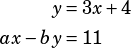In the system of equations above,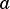andare constants. If the system has no solutions, what is the value of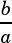?

A)
B)
C)
D)
2

In the-plane, a triangle is formed with vertices at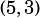,, and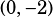. What is the area of the triangle?

3A square is formed in the-plane by connecting four points: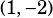,,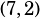, and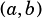, whereandare constants. What is the value of?

4Which of the following equations, when graphed in the-plane, does not contain any points in Quadrant IV?

A)
B)
C)
D)
5A certain parabola has the equation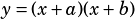, whereandare constants. In terms ofand, what is the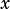-value of the parabola's vertex?
A)
B)
C)
D)

Section progress